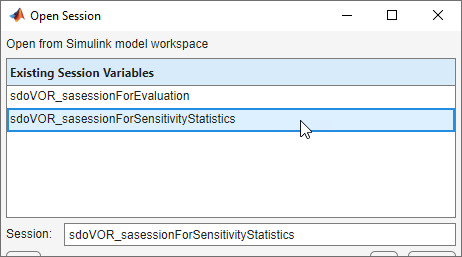Generate MATLAB Code for Sensitivity Analysis Statistics to Identify Key Parameters (GUI)

This example shows how to automatically generate a MATLAB function to solve a Sensitivity Analysis statistics problem. You use the Sensitivity Analyzer to define a sensitivity statistics problem for a model of the body's vestibulo-ocular reflex, and generate MATLAB® code to solve this statistics problem.

Vestibulo-Ocular Reflex Sensitivity Statistics Problem

The Identify Key Parameters for Estimation (GUI) example shows how to use the Sensitivity Analyzer to compute sensitivity statistics for different parameter values in a model of the body's vestibulo-ocular reflex. In this example, we load a preconfigured Sensitivity Analyzer session based on that example.

Open the Sensitivity Analyzer for the sdoVOR model:

ssatool('sdoVOR')

In the Sensitivity Analyzer, click Open Session and Open from model workspace. Open session sdoVOR_sasessionForSensitivityStatistics.This opens a preconfigured session in the Sensitivity Analyzer.Generate MATLAB Code

From the Compute Statistics list, select Generate MATLAB Function.The generated code is added to the MATLAB editor as an unsaved MATLAB function.Examine the generated code. Significant portions are:

• Statistics Analysis Variables - Specify the inputs and outputs, to determine which inputs have the most influence on outputs.

• Statistics Options - Specify the types of analyses to be computed.

• Compute Statistics - Solve the sensitivity statistics problem using the sdo.analyze command.

Select Save from the MATLAB editor to save the generated function.

Run Generated Code

Run the generated function.The computation shows the result of analyzing which inputs have the most influence on the output. For example, the Correlation field shows that the Gain parameter has the largest magnitude correlation with the output, and in a negative direction, meaning that when Gain increases, the output decreases.

Modify the Generated Code

You can:

• Modify the specified input and output variables.

• Modify the options to change the types of analyses computed.

Related Topics